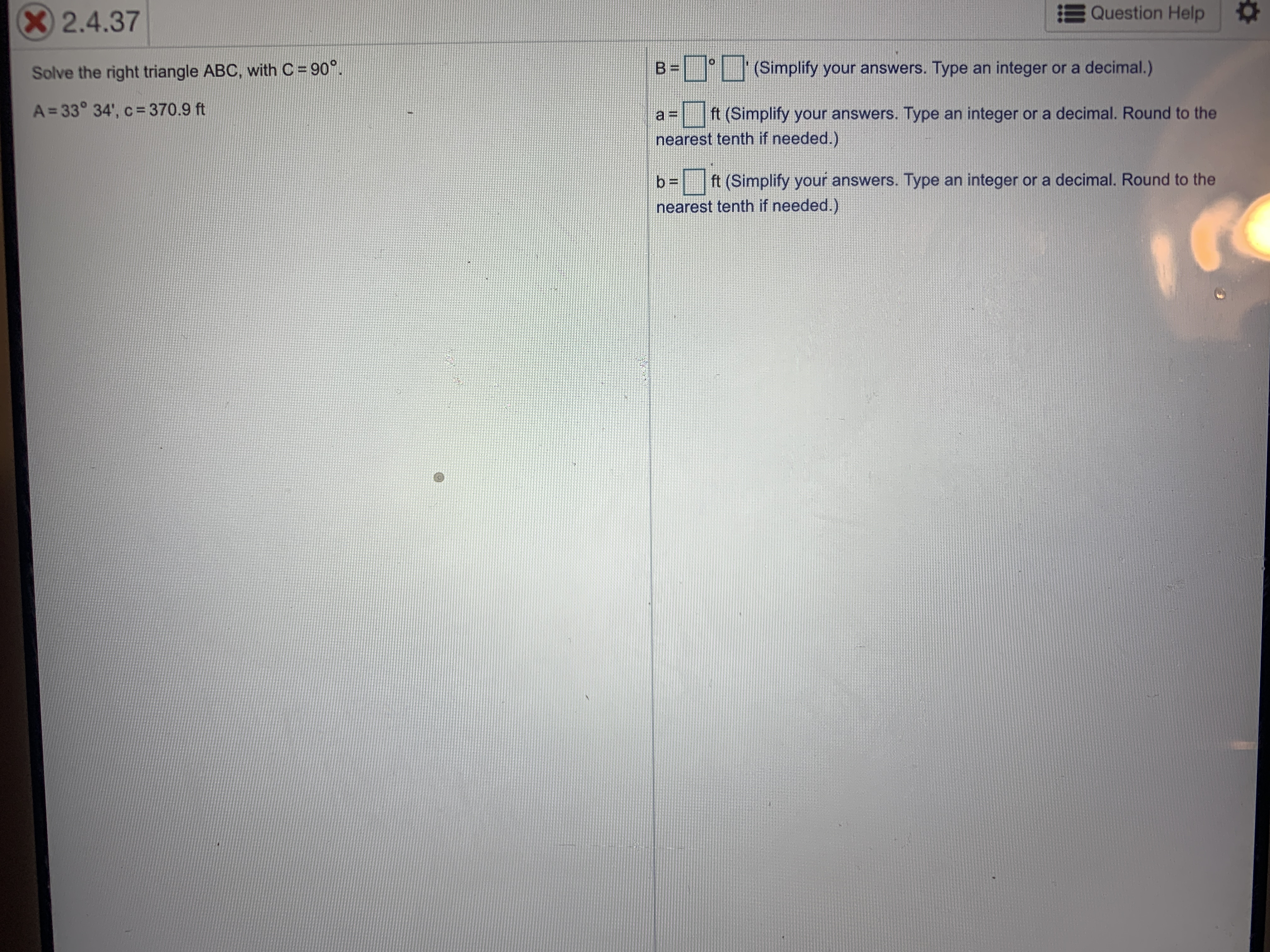# X 2.4.37Question HelpSolve the right triangle ABC, with C 90°B =в -(Simplify your answers. Type an integer or a decimal.)A=33° 34', c=370.9 ftанft (Simplify your answers. Type an integer or a decimal. Round to thenearest tenth if needed.)b=ft (Simplify your answers. Type an integer or a decimal. Round to thenearest tenth if needed.)

Questionhelp_outlineImage TranscriptioncloseX 2.4.37 Question Help Solve the right triangle ABC, with C 90° B = в - (Simplify your answers. Type an integer or a decimal.) A=33° 34', c=370.9 ft ан ft (Simplify your answers. Type an integer or a decimal. Round to the nearest tenth if needed.) b= ft (Simplify your answers. Type an integer or a decimal. Round to the nearest tenth if needed.) fullscreen
check_circleExpert Solution
Step 1

Sum of the acute angles in right triangle = 90 degree.

One angle is A= 33 degree 34'
So, B=90 degree - (33 degree 34')=56 degree 26'

Step 2

Use adjacent/hypotenuse=cos theta to find a.

Used calcu...

### Want to see the full answer?

See Solution

#### Want to see this answer and more?

Solutions are written by subject experts who are available 24/7. Questions are typically answered within 1 hour*

See Solution
*Response times may vary by subject and question
Tagged in

### Trigonometry